# Mixed Numbers and Improper Fractions, Explained

They may look funky at first, but we promise mixed numbers and improper fractions don’t have to throw you for a loop – let’s learn about them together!Author
Taylor HartleyExpert Reviewer

Published: August 24, 2023# Mixed Numbers and Improper Fractions, Explained

They may look funky at first, but we promise mixed numbers and improper fractions don’t have to throw you for a loop – let’s learn about them together!Author
Taylor HartleyExpert Reviewer

Published: August 24, 2023# Mixed Numbers and Improper Fractions, Explained

They may look funky at first, but we promise mixed numbers and improper fractions don’t have to throw you for a loop – let’s learn about them together!Author
Taylor HartleyExpert Reviewer

Published: August 24, 2023Key takeaways

• Mixed numbers and improper fractions are different ways to write the same thing – Mixed numbers include whole numbers and fractions, while improper fractions don’t have any whole numbers.
• It’s easy to change improper fractions into mixed numbers – But you have to understand division and key terms like numerator and denominator.
• Decimals are a different way of expressing mixed numbers – You can also easily turn mixed numbers into decimals. Don’t worry, we’ll show you how!

Platform 9 ¾. The Lion King 1 ½. You’ve probably seen mixed numbers before, but you might not know exactly what they mean or how they’re created. Now, your teacher’s asking you to turn improper fractions into mixed numbers, mixed numbers into improper fractions, and mixed numbers into decimals – all at one time!

Don’t worry: mixed numbers aren’t nearly as wonky as they look. Today, we’ll take a look at what mixed numbers are, how to convert improper fractions into mixed numbers and vice versa, and what to do when your teacher asks, “Can you make that into a decimal for me?”

Let’s dive in.

## What are mixed numbers?We know what numbers are, right? And we know that when you “mix” things together, you’re bringing together two things that may be related, but are not entirely the same thing. So think of a “mixed number” as a combination of both a whole number and a fraction, or a part of a whole.

Let’s think about it another way. Say you’re having a pizza party. Your mom orders several pizzas so you and your friends can each have more than one slice. If your friends eat one whole pizza and then half of another pizza, you’ve eaten 1 ½ pizzas. That’s a mixed number!

### Important terms to know• Whole number – Whole numbers are complete. Going back to our pizza analogy, one pizza is the whole number, ‘1’.
• Fraction – A fraction represents a part of a whole. It’s made up of two numbers, a numerator, the number on top, and a denominator, the number on the bottom.
• Numerator – This is the number on the top of the fraction, and it’s actually part of the whole number. So, if there are 4 pieces of pizza and you take 1, you’ve taken ¼ of the whole.
• Denominator – This is the number on the bottom of the fraction, and it represents the whole number. So, if your pizza has three slices, the denominator is 3.
• Improper fraction – This happens when the numerator is bigger than the denominator. For example, if you have three half-slices, we write it as 3/2.

## How to convert improper fractions to mixed numbersSometimes, you’re going to be given an improper fraction, like 14/6. Your teacher will then ask you, “convert this into a mixed number.” But exactly how can you do that? Let’s walk through the process step by step.

### Step 1: DivideRemember, an improper fraction is really just a division problem in disguise. Let’s stay with our improper fraction 14/6 and stick with our pizza analogy. A whole pizza is made up of 6 slices, but you have 14. So how many pizzas do you really have here?

First, divide 14 by 6. 6 goes into 14 two times, so 2 (the quotient) is going to be your whole number.

Now, you still have 2 left over, because 6 times 2 equals 12, not 14.

### Step 2: Rewrite your quotient as a whole number with the remainder as a fractionThe first part of your quotient – or the answer to Step 1 – becomes the whole number part of the mixed number: 2.

Your remainder, 2, becomes the numerator in the fraction part of your mixed number. Now, the denominator is still 6, because that’s how many “parts” are in the whole, or in our example, how many slices make a whole pizza.Your answer is a mix of your whole number and your fraction (or how many pizzas you have and how many extra slices are leftover).

So 2 is the whole number. 2/6 is the fraction. And 2 goes into six 3 times, so you can simplify 2/6 to be ⅓.

Your mixed number is 2 ⅓.

## How to convert mixed numbers to improper fractionsLet’s do the reverse now. This is like counting up all the slices you have and saying how many slices you have over how many pizzas you can make.

Let’s say you have 1 ¾ of pizzas.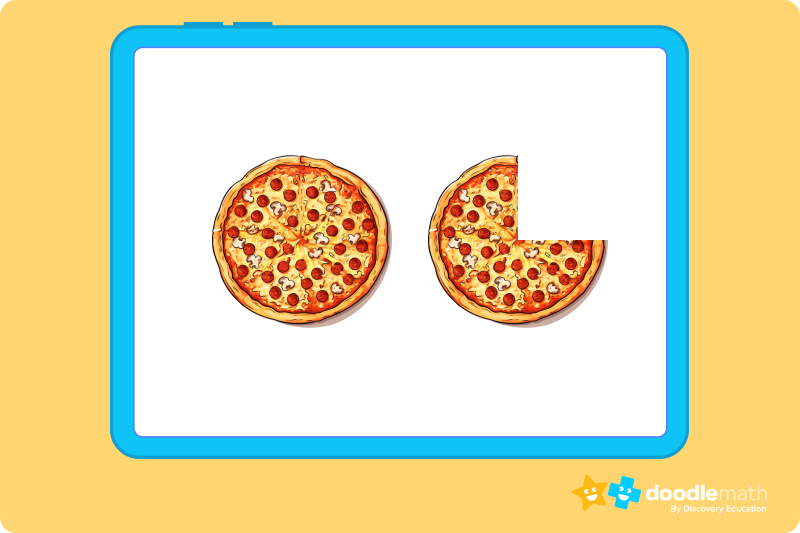### Step 1: Multiply the whole number by the fraction’s denominatorIn our example, you multiply 1 (your whole number) by 4 (the fraction’s denominator). You get ‘4’.

### Step 2: Add the result from step 1 to the fraction’s numeratorYou add 4 (the result from step 1) to 3 (the fraction’s numerator) and get ‘7’.

### Step 3: Write down your answer as the numerator and keep the fraction’s original denominator‘7’ becomes your new numerator, and the denominator stays the same, which is ‘4’. So, 1 3/4 as an improper fraction is 7/4.

## How to add mixed numbersSometimes, your teacher may ask you to add mixed numbers together. Don’t panic! It isn’t nearly as messy as it looks.

We will stick with our pizza analogy to help us visualize our problem.

Let’s imagine we have two picnic tables of pizza. One table has 5 whole pizzas and 1/3 of another pizza, and the second table has 7 whole pizzas and another 1/3 of a pizza.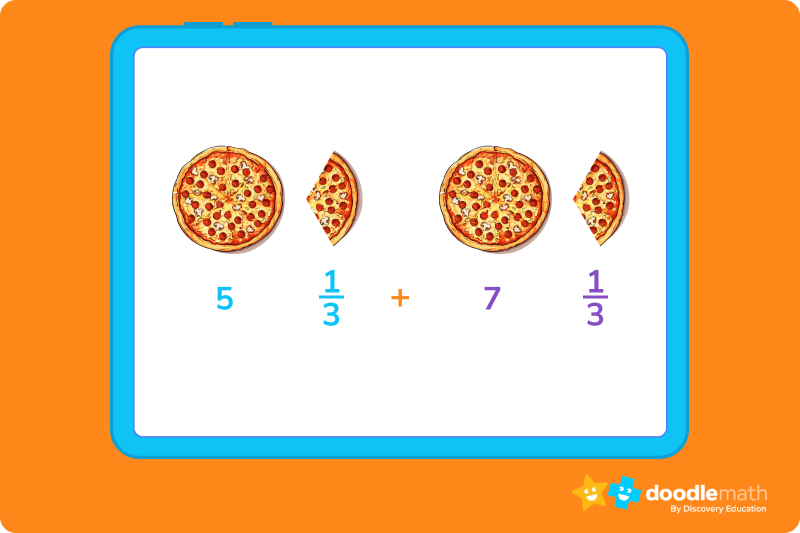5 ⅓ + 7 ⅓ = ?

### Step 1: Change your mixed numbers into improper fractionsThe fractions in each of these problems tell us that there are 3 pieces per pizza.  First, let’s figure out how many individual slices we have on each table.

5 pizzas × 3 slices per piece = 15 pieces on the first table, along with the 1 slice from 1/3. That’s 16 slices.

On the second table, we have 7 pizzas with 3 slices each, so 7 pizzas × 3 slices = 21 slices, plus the 1 slice from ⅓, so 22 slices.

So, 5 ⅓ = 16/3 as an improper fraction.

And 7 ⅓ = 22/3 as an improper fraction.

### Step 2: Add our improper fractionsNow we know that there are 16 slices on one table and 22 slices on the other. Since, all our slices are ⅓ of a pizza, so we can just add them together.

Numerators: 16 + 22 = 38

Improper fraction: 38/3

### Step 3: Convert your improper fraction to a mixed numberWe end up with 38 slices, which is a lot of slices! So let’s turn them back into pizzas or a mixed number. We know that 3 slices make up one whole pizza, so divide 38 slices by 3 to see we have12 whole pizzas and 2 leftover slices (38 3 = 12 Remainder 2).

So, the answer is 12 2/3 pizzas.

## Converting mixed numbers to decimalsEven though they look different, mixed numbers and decimals are alike. They both have a whole number and a portion of a whole. A decimal number, like 2.25, has a whole number (2) and a little part after the decimal (.25). A mixed number, like 2 1/4, also has a whole number (2) and a little part (1/4), written as a fraction instead of a decimal. They’re just different ways of showing the same thing!

To change a mixed number into a decimal, we follow these steps.

Let’s try this with an example: Change 3 1/4 into a decimal.

### Step 1: Keep the whole number (3) and set it asideThe whole number is 3.

### Step 2: Now, convert the fraction (the slices of the pizza) into a decimal.We do this by dividing the numerator (1) by the denominator (4)

Our decimal is 0.25

### Step 3: Finally, we put all our slices back together to get 3 whole pizzas and 0.25 of a pizzaSo 3 ¼ pizzas is the same as 3.25 pizzas in decimal form.

## Let’s practice together!You’ve done a great job following along! Now it’s time for the best part – practice. The more we do it, the better we get! Remember, we’re going to treat each problem just like we’re figuring out our pizzas. Let’s get started!

### 1. Convert the improper fraction 7/3 to a mixed numberLet’s go through it step by step.

1. Divide the numerator (7) by the denominator (3).

We know that 3 goes into 7 two times, so 7 ÷ 3 = 2 with a remainder of 1.

2. The whole number part of the mixed number is the quotient from step 1, which is 2.

Whole number: 2

3. The numerator of the fractional part is the remainder from step 1, which is 1.

Numerator: 1

4. The denominator remains the same.

Denominator: 3

Therefore, the mixed number representation of 7/3 is 2 1/3.

### 2. Convert the improper fraction 10/4 to a mixed numberLet’s break it down:

1. Divide the numerator (10) by the denominator (4).

10 ÷ 4 = 2, with a remainder of 2.

2. The whole number part of the mixed number is the quotient from step 1, which is 2.

Whole number: 2

3. The numerator of the fractional part is the remainder from step 1, which is 2.

Numerator: 2

4. The denominator remains the same.

Denominator: 4

Therefore, the mixed number representation of 10/4 is 2 2/4, or if we simplify 2 2/4, it’s 2 ½.

### 3. Convert the improper fraction 13/5 to a mixed numberHere we go again!

1. Divide the numerator (13) by the denominator (5).

13 ÷ 5 = 2 with a remainder of 3

2. The whole number part of the mixed number is the quotient from step 1, which is 2.

Whole number: 2

3. The numerator of the fractional part is the remainder from step 1, which is 3.

Numerator: 3

4. The denominator remains the same.

Denominator:

Therefore, the mixed number representation of 13/5 is 2 3/5.

### 4. Convert the mixed number 2 3/4 to a decimalNow we’re switching it up! Let’s use our steps to walk through this:

1. Start with the whole number part, which is 2. Write it down as a whole number.

Whole number: 2

2. Next, convert the fractional part to a decimal. Divide the numerator (3) by the denominator (4).

3 ÷ 4 = 0.75

3. Write down the decimal obtained from step 2.

Decimal: 0.75

4. Combine the whole number and the decimal obtained to get the final answer.

Therefore, the decimal representation of the mixed number 2 3/4 is 2.75.

### 5. Add the mixed numbers 1 3/4 + 2 1/2Let’s practice together.

1. Change your mixed numbers into improper fractions.

For 1 ¾ Multiply the whole number (1) by your denominator (4) and add the numerator (3).

1×4+3= 7

For 2 ½ multiply the whole number (2) by your denominator (2) and add the numerator (1).

2×2+1= 5

To do this, find the common denominator for 7/4 and 5/2, which is 4. Rewrite both fractions with the common denominator.

Multiply the numerator and denominator in your second fraction (5/2)  by 2 making 10/4. Now that the denominators are the same, add the numerators.

10/4 + 7/4 = 17/4

3. Convert your improper fraction to a mixed number.

First, divide the numerator (17) by the denominator (4) giving you a quotient of 4. This is your whole number. The remainder (1) is your new numerator.

Ready to give it a go?

Great job learning about fractions and mixed numbers. Now, let’s practice. Practicing helps us get better and lets us show what we can do. Remember to tackle each problem just like we’ve learned. Let’s go!

## Parent Guide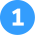How did we get here?

1. Divide the numerator (9) by the denominator (2).

9 ÷ 2 = 4, with 1 left over (remainder).

2. The whole number part of the mixed number is the quotient from step  1, which is 4.

Whole number: 4

3. The numerator of the fraction is the remainder from step 1, which is 1.

Numerator: 1

4. The denominator remains the same.

Denominator: 2

Therefore, the improper fraction 9/2 is 4 ½ as a mixed number.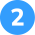How did we get here?

1. Multiply the whole number (5) by the denominator of the fraction (4).

5 x 4 = 20

2. Add this result to the numerator of the fraction (1).

20 + 1 = 21

3. This sum becomes your new numerator, and the denominator stays the same.

Numerator: 21

Denominator: 4

So, the improper fraction representation of 5 1/4 is 21/4.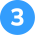Here’s how we do it.

1. Keep the whole number part, which is 3.

Whole number: 3

2. Convert the fractional part (1/3) into a decimal by dividing the numerator (1) by the denominator (1). This gives you 0.33.

Decimal fraction: 0.33

3. Add 0.33 to 1, so you get 1.33.

So, 1 1/3 as a decimal is 1.33.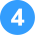How did we get here?

1. Keep the whole number part, which is 2.

Whole number: 2.

2. Convert the fractional part (1/2) into a decimal by dividing the numerator (1) by the denominator (2). This gives you 0.5

Decimal fraction: 0.75.

3. Add 0..5 to 2, so you get 2.05.

So, 2 1/2 as a decimal is 2.05.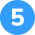Let’s go through the process.

1. Convert these mixed numbers into improper fractions.

2 2/3 becomes 8/3 and 1 1/3 becomes 4/3.

2. Now, let’s add the fractions. Since the denominators are already the same (which is 3), we just add the numerators.

This gives us (8 + 4)/3, which simplifies to 12/3.

3. Lastly, let’s convert the resulting improper fraction back into a mixed number.

This gives you 4.

So, 2 2/3 + 1 1/3 = 4.

## FAQs about math strategies for kidsWe understand that diving into new information can sometimes be overwhelming, and questions often arise. That’s why we’ve meticulously crafted these FAQs, based on real questions from students and parents. We’ve got you covered!

A mixed number is a mix of a whole number and a fraction. It’s like having a whole item and a part of another item together. Some examples of mixed numbers are 10 ½, 5 1/3, and 7/34.

In an improper fraction, the denominator is greater than (or equal to) the numerator. Examples of improper fractions are 9/5, 8/7, and 10/4.

Mixed numbers and improper fractions are different ways to write the same amount. In an improper fraction, the denominator tells us the size of the parts and the numerator tells us the number of parts. In a mixed number, the denominator tells us the size of the parts and the whole number and numerator in the fraction tell us the number of parts together.

First, multiply the whole number by the denominator. Next, add the numerator to this result. Then, write your new fraction with the answer from the second step. The denominator remains the same.

First, divide the numerator by the denominator. Next, use the result (quotient) as the whole number. Then, use the remainder as the numerator of the fraction. The denominator remains the same.## Related Posts

Title 1

Title 2

Title 3

Lesson credits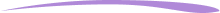Taylor Hartley

Taylor Hartley is an author and an English teacher based in Charlotte, North Carolina. When she's not writing, you can find her on the rowing machine or lost in a good novel.

Jill Padfield has 7 years of experience teaching high school mathematics, ranging from Alegra 1 to AP Calculas. She is currently working as a Business Analyst, working to improve services for Veterans while earning a masters degree in business administration.

Taylor Hartley

Taylor Hartley is an author and an English teacher based in Charlotte, North Carolina. When she's not writing, you can find her on the rowing machine or lost in a good novel.

Jill Padfield has 7 years of experience teaching high school mathematics, ranging from Alegra 1 to AP Calculas. She is currently working as a Business Analyst, working to improve services for Veterans while earning a masters degree in business administration.# Are you a parent, teacher or student?

Are you a parent or teacher?

## Hi there!

Book a chat with our team